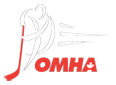# AGM registration (Petrolia Minor Hockey)

PrintAGM registration

AGM 2016-2017

REGISTRATION

Registration starts Apr 15 to May 31

Late fee starts June 1st to June 30th of \$150 and placed on a wait list.

2016/17 = 309 registered

2015/16 = 317 registered

2014/15 = 306 registered

Tim bit            Atom Midget

2016/17 = 44 2016/17 = 50 2016/17 = 51

2015/16 = 49 2015/16 = 50 2015/16 = 47

2014/15 = 44 2015/14 = 44 2014/15 = 49

Tyke PeeWee

2016/17 = 46 2016/17 = 32

2015/16 = 41 2015/16 = 38

2014/15 = 41 2014/15 = 42

Novice Bantam

2016/17 = 41 2016/17 = 45

2015/16 = 48 2015/16 = 44

2014/15 = 45 2014/15 = 41Latest News Hockey Labs Tournaments Coach Resources Trainer Resources